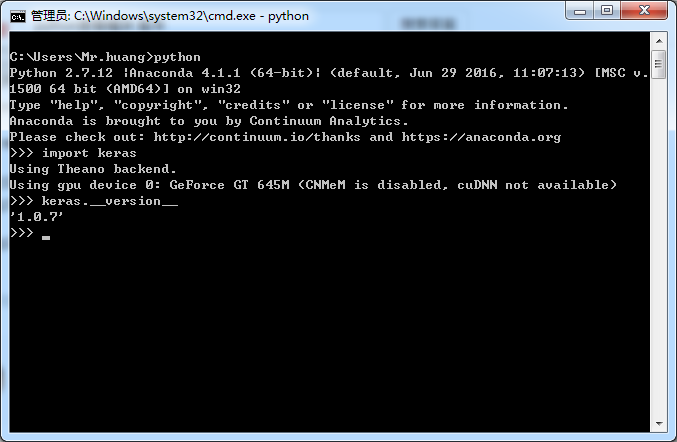2016-08-14 15:58

# 深度学习框架 keras 如何实现 AutoEncoder ？

1.从文本文档中导入样本(可选)
2.利用自动编码器取出特征(必须)
3.把编码得出的特征保存到一个文本文档中(说明怎么取编码得到的特征也行)• 写回答
• 关注问题
• 收藏
• 邀请回答

#### 3条回答默认 最新

•Valtava 2016-08-16 15:42
已采纳

仔细读一下里面的例子就可以了。
假设你的input 叫做 in_data, 中间特征层叫做 code, 最终输出叫做out_data，
那么训练完成之后只需要encoder = Model(input=in_data, output=code)就可以构建encoder部分（特征提取），
然后features = encoder.predict(X_train)就可以得到特征 （假设你的输入叫做X_train）

得到的特征(上述features)变量是个numpy数组，你可以随意把这个数组存到本地（npy, pickle, 循环遍历等方法看你个人喜好。。。）

理论上，样本多了当然比少了好，而且本来autoencoder就不需要label，所有数据全用了在分类问题里都不能算作弊（毕竟没有用label）

已采纳该答案
打赏 评论
•Valtava 2016-08-21 09:01
``````from   keras.layers import Dense, Input
from   keras.models import Model
import numpy as np

def main():
X_train = np.array([[1,0,0,0], [0,1,0,0], [0,0,1,0], [0,0,0,1]])

print "building model .."
in_code = Input(shape=(4,))
feature_code = Dense(3, activation='linear')(in_code)
out_code = Dense(4, activation='relu')(feature_code)
model = Model(input=in_code, output=out_code)

print "compiling and training .."
model.compile(optimizer='sgd', loss='mse')
for i in range(1000):
model.fit(X_train, X_train, verbose=0, batch_size=4)

print "checking network .."
recons = model.predict(X_train, verbose=0)
print "reconstructed data at output:\n", recons

print "extracting feature .."
feature_extractor = Model(input=in_code, output=feature_code)
features = feature_extractor.predict(X_train, verbose=0)
print "feature for input(with dimensionality 3):\n", features

if __name__=='__main__':
main()
``````

结果如下（初值随机，因此结果不是总相同）

`````` building model ..
compiling and training ..
checking network ..
reconstructed data at output:
[[  0.00000000e+00   6.50808215e-06   1.13397837e-05   0.00000000e+00]
[  0.00000000e+00   9.99989569e-01   0.00000000e+00   6.85453415e-06]
[  0.00000000e+00   0.00000000e+00   9.99984086e-01   0.00000000e+00]
[  1.37090683e-06   3.72529030e-06   0.00000000e+00   9.99991655e-01]]
extracting feature ..
feature for input(with dimensionality 3):
[[-0.00143426  0.36764431  0.17566341]
[-0.82009792  0.14263746  0.32771838]
[ 0.86068124  0.05564899  1.00661278]
[-0.44917884 -0.44360706 -0.93941289]]
``````
打赏 评论